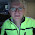## July 25, 2014

### Speeding up postcode queries

By John Hyde

In an article on Hex Central, Mike Lewis showed how to calculate the distance between any two British postcodes. Here’s a tip for speeding up the process.

The calculation that Mike demonstrated is a simple application of Pythagoras’ theorem. You start by getting the grid references (that is, the x, y co-ordinates) of the two postcodes. Next, add the sums of the squares of the x and y distances between them. Finally, take the square root of the value thus obtained. That final figure is the straight line distance between the two points.

My tip is simply to omit the calculation of the square root. So, instead of working with the actual distance, you work with the square of that distance.

As an example, let’s suppose you want to sort a series of postcode pairs into descending order of distance apart. You omit the calculation of the square root, which means that you will in fact be sorting by the square of the distance apart. The result will still be correct.

Similarly, if you want to find all postcodes within a given radius of a fixed point, you omit the square root calculation, and compare the distances with the square of the target radius. Again, this will give the correct result.

Since the calculation of the square root is likely to be the most time-consuming part of the process, leaving it out should speed things up considerably.

1.John, thank you for this useful tip. I've added a link to it from the orginal article.

2.You are very welcome.

The reason I am looking at this subject is because I am writing a Drupal module for location-based search in the UK.

3.Another tip for a fast query getting points within R miles of another point using grid references.

Don't get the points in a circle of radius R. Instead, define a square box that encloses the circle. The sides will be 2R. This can be a very fast database query.

Get all the points in the box from the database and into your program. You can then use a faster compiled language to remove the corner points that are in the square but not in the circle. Or even leave them in if you are not too fastidious and your application isOK with this.

1.Another excellent tip, John. Thank you. So you are eliminating the calculation of the sum of the squares, and instead just comparing the absolute value of the difference in the eastings with R, and ditto with the northings. That would certainly be faster.

Regarding the use of a compiled language to remove the corner points, another option would be to get the first set of points (those that are within the sqaure) into an intermediate result set, and then apply the original query just to that result set, which would be considerably smaller than the entire population of points.

But I also like the idea of the application not being too fastidious on this point. Getting the points that are in the square rather than in the circle will be a good enough approximation in many cases.